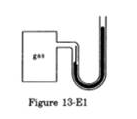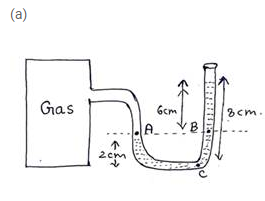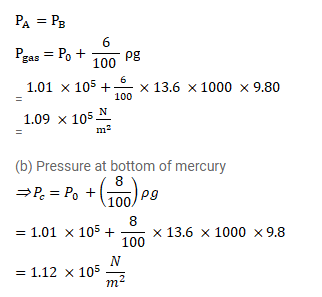# The height of mercury surfaces in the two arms of the manometer shown in figure (13-E1)

Question:

The height of mercury surfaces in the two arms of the manometer shown in figure (13-E1) are $2 \mathrm{~cm}$ and $8 \mathrm{~cm}$. Atmospheric pressure $=1.01 \times 10^{5} \mathrm{~N} / \mathrm{m}^{2}$. Find

(a) the pressure of the gas in the cylinder and

(b) the pressure of mercury at the bottom of the $U$ tube.Solution: